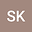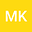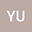Strang Time-Splitting Technique for the Generalized Rosenau-RLW Equation
•••• Selcuk KUTLUAY,
• Melike Karta,
• Yusuf UCAR
Selcuk KUTLUAY
Inonu University
Author ProfileMelike Karta
Agri Ibrahim Cecen Universitesi
Author ProfileYusuf UCAR
Inonu University
Author Profile## Abstract

In this paper, a numerical scheme based on quintic B-spline collocation method using the Strang splitting technique is presented for the solution of the generalized Rosenau-RLW equation given by appropriate initial-boundary values. For our purpose, firstly the problem is split into two subproblems such that each one includes the derivative in the direction of time. Secondly, each subproblem using collocation finite element method with quintic B-splines for spatial integration is reduced to a system of ordinary differential equations (ODEs). Then, the resulting ODEs for time integration are solved using the Strang time-splitting technique with the second order via the usual Runge-Kutta (RK-4) algorithm with the fourth order. To measure the accuracy and efficiency of the present scheme, a model problem with an exact solution is taken into consideration and investigated for various values of the parameter p. The L₂ and L_{∞} errors with the invariants of discrete mass Q and discrete energy E have been computed and given a comparison with other ones found in the literature. The convergence order of the present numerical scheme has also been computed. Furthermore, the stability analysis of the scheme is numerically examined.